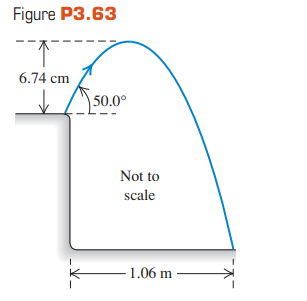### Solution Found!# Answer: A grasshopper leaps into the air from the edge of

Chapter 3, Problem 63P

#### (choose chapter or problem)

Get Unlimited Answers! Check out our subscriptions

QUESTION:

A grasshopper leaps into the air from the edge of a vertical cliff, as shown in Fig. P3.63. Use information from the figure to find (a) the initial speed of the grasshopper and (b) the height of the cliff.Step 1 of 5

(a)  the initial speed of the grasshopper

Let us consider vertical component of velocity,where,as it reaches max height, its final velocity will be zero,Initial velocity=v sin 50,

distance s =  6.74 cm = 0.0674 m

Acceleration , a = g =Substituting given data in above equation,v = 1.5 m/s

Therefore, the initial velocity of the grasshopper is  1.5 m/s.

Become a subscriber and get unlimited answers!